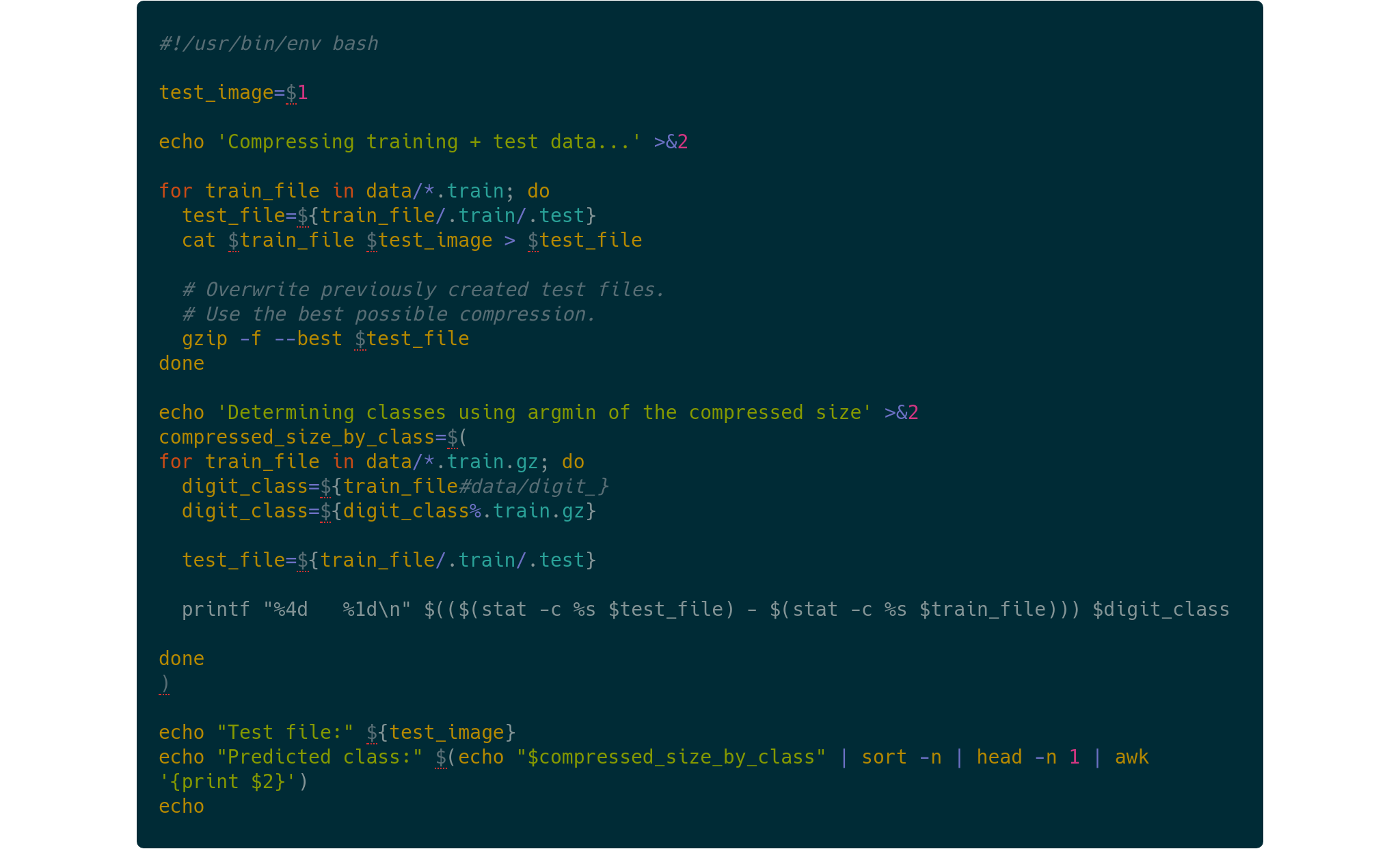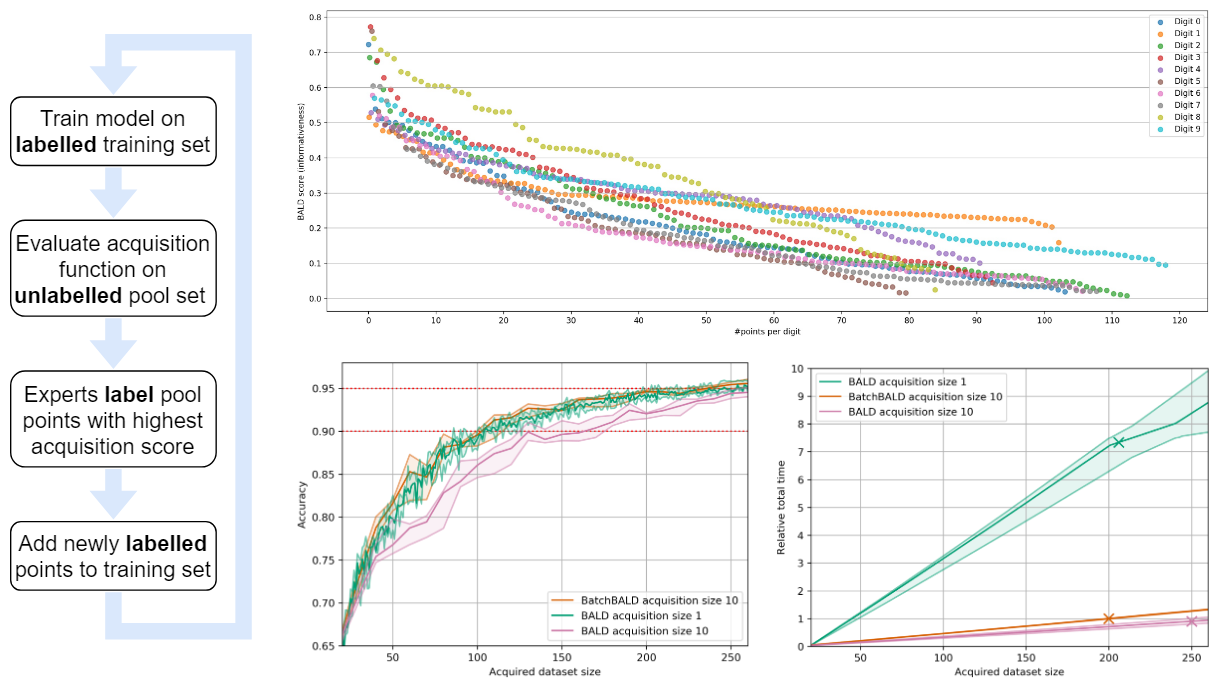research blog

• Stirling's Approximation for Binomial Coefficients

In MacKay (2003) on page 2, the following straightforward approximation for a binomial coefficient is introduced: $\begin{equation} \log \binom{N}{r} \simeq(N-r) \log \frac{N}{N-r}+r \log \frac{N}{r}. \end{equation}$ The derivation in the book is short but not very intuitive although it feels like it should be. Information theory would be the likely candidate to provide intuitions. But information-theoretic quantities like entropies do not apply to fixed observations, only random variables, or do they?

• Better intuition for information theory

The following blog post is based on Yeung’s beautiful paper “A new outlook on Shannon’s information measures”: it shows how we can use concepts from set theory, like unions, intersections and differences, to capture information-theoretic expressions in an intuitive form that is also correct.

The paper shows one can indeed construct a signed measure that consistently maps the sets we intuitively construct to their information-theoretic counterparts.

This can help develop new intuitions and insights when solving problems using information theory and inform new research. In particular, our paper “BatchBALD: Efficient and Diverse Batch Acquisition for Deep Bayesian Active Learning” was informed by such insights.

• MNIST by ziptl;dr: We can use compression algorithms (like the well-known zip file compression) for machine learning purposes, specifically for classifying hand-written digits (MNIST). Code available: https://github.com/BlackHC/mnist_by_zip.

• Human in the Loop: Deep Learning without Wasteful LabellingIn Active Learning we use a “human in the loop” approach to data labelling, reducing the amount of data that needs to be labelled drastically, and making machine learning applicable when labelling costs would be too high otherwise. In our paper  we present BatchBALD: a new practical method for choosing batches of informative points in Deep Active Learning which avoids labelling redundancies that plague existing methods. Our approach is based on information theory and expands on useful intuitions. We have also made our implementation available on GitHub at https://github.com/BlackHC/BatchBALD.